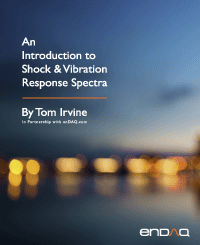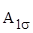Sinusoidal Vibration
By Tom Irvine
SECTION 1

# Descriptive Statistics

A sine function is characterized by its frequency and amplitude. The frequency is the inverse of the period, where the period is the duration of one cycle. The amplitude may be represented by any of the three parameters shown on the right-hand-side. The zero-to-peak and RMS amplitudes for sinusoidal oscillations are related by

Note that

whereis the standard deviation

The RMS value is thus equation to the standard deviation for the case of zero mean. A pure sine function always has zero mean.

A sine function and its corresponding histogram are shown in Figure 1.2. The histogram divides the amplitude points into bins and then counts the points in each bin. The number of points in each bin are then plotted as a bar graph. Some of the histograms in this document are turned 90 degrees clockwise and shown adjacent to the corresponding time history for educational purposes.

A sine function has a bathtub histogram. The amplitude tends to dwell at either the peak or valley with a fairly rapid transition in between. Histograms are important for fatigue analysis.

Now let x(t) be a sinusoidal displacement function. The displacement equation is

where

X     is the zero-to-peak amplitude

The corresponding velocity v(t) is

The acceleration a(t) is

Table 1.1. Peak Values Referenced to Peak Displacement
Parameter Value
displacement X
velocity w X
acceleration w2 X
Table 1.2. Peak Values Referenced to Peak Acceleration
Parameter Value
acceleration A
velocity A / w
displacement A / w2

Let A be the peak acceleration.

Conversely,

SECTION 2

## Sine Testing

Table 1.2 has enormous consequences for vibration testing. Commercial shaker tables, such as those found in Figure 4.9 and Figure 4.10, are typically limited to a few inches peak-to-peak displacement unless they are large-scale tables specifically designed for earthquake simulation. Vibration tests are usually specified in terms of acceleration. The test specification starting frequency is typically 10 to 20 Hz to limit the displacement.

SECTION 3
Sample Sine Calculations

Astronaut Michael Collins wrote :

The first stage of the Titan II vibrated longitudinally, so that someone riding on it would be bounced up and down as if on a pogo stick. The vibration was at a relatively high frequency, about 11 cycles per second, with an amplitude of plus or minus 5 Gs in the worst case.

The equivalent angular frequency for 11 Hz is 69.1 rad/sec. Calculate the corresponding displacement X using equation (1.7).

M.J. Griffin  cited a study where 5% of the passengers on a ship experienced vomiting while the ship underwent a 0.2 m/sec^2 acceleration amplitude during a 3 hour. Assume a 0.2 Hz frequency (1.26 rad/sec^2). Calculate the displacement.

SECTION 4
Beat Frequency EffectFigure 4.1. Channel Beam Free Vibration Response at Free End to Force Impulse

Consider the case of two closely-space vibration frequencies generating sound. The envelope of the two signals oscillates at a frequency which is the differences of the two frequencies. This beat frequency is detected by the human hearing and brain system, but it is a psychoacoustic effect which does not affect the mechanical hardware.

A channel beam was mounted in a cantilever configuration. The free end was struck with an impulse hammer. The resulting acceleration time history at the free end is shown in Figure 4.1. A synthesized damped sine curve-fit yielded natural frequencies at 583 and 691 Hz. The beat frequency is the difference which is 108 Hz.